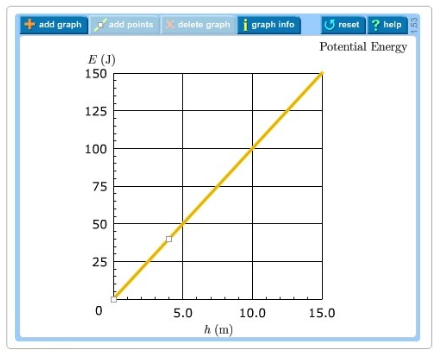# Problem: A 1.00kg ball is thrown directly upward with an initial speed of 16.0 m/s.A graph of the ball's gravitational potential energy vs. height, for an arbitrary initial velocity, is given in Part A. The zero point of gravitational potential energy is located at the height at which the ball leaves the thrower's hand.For this problem, take g = 10 m/s2 as the acceleration due to gravity.Draw a line on the graph representing the total energy of the ball.

###### FREE Expert Solution

Gravitational potential energy:

$\overline{)\begin{array}{rcl}{\mathbf{U}}& {\mathbf{=}}& \mathbf{m}\mathbf{g}\mathbf{h}\end{array}}$

Maximum height:

$\overline{){{\mathbit{H}}}_{\mathbit{m}\mathbit{a}\mathbit{x}}{\mathbf{=}}\frac{{{\mathbit{v}}_{\mathbf{0}}}^{\mathbf{2}}}{\mathbf{2}\mathbit{g}}}$

h = v02/2g = 16.02/(2 × 10) = 12.8 m

Umax = mgh = 1.00 × 10 × 12.8 = 128 J

97% (236 ratings)###### Problem Details

A 1.00kg ball is thrown directly upward with an initial speed of 16.0 m/s.

A graph of the ball's gravitational potential energy vs. height, for an arbitrary initial velocity, is given in Part A. The zero point of gravitational potential energy is located at the height at which the ball leaves the thrower's hand.

For this problem, take g = 10 m/s2 as the acceleration due to gravity.

Draw a line on the graph representing the total energy of the ball.